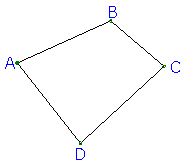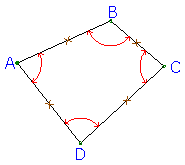## 1. Traverse

A traverse is a series of connected lines whose lengths and adjacent angular relationships are measured. Traverses are used to determine horizontal positions, relative locations, and areas. A traditional traverse is two-dimensional in a horizontal plane. As Total Station Instrument technology evolved, more and more traverse data was collected in all three dimensions, incorporating elevation. This topic will address only two-dimensional horizontal traverses although many of the concepts are easily adopted to three-dimensions.

## 2. Configuration

Configuration is the physical network design of a traverse; it can be either a loop or a link. A loop traverse closes back on itself forming a polygon while a link traverse begins and ends on two different points, Figure A-1.Figure A-1 Traverse Configurations

## 3. Type

Type is defined mathematically. A closed traverse has sufficient measurements to enable one or more math checks which facilitate tracking down errors. An open traverse has just enough measurements to compute point positions, but not enough to check mathematical conditions.

For example, the loop traverse in Figure A-2 is mathematically closed because all four distances and angles have been measured. Each traverse point's relative position is fixed with respect to the others. One of the traverse's mathematical conditions which can be checked is that the angles should sum exactly to 360°.Figure A-2 Closed Loop Traverse

The loop traverse in Figure A-3(a) has only distances measured, no angles. It is open because its polygonal configuration isn't fixed by distances alone. The traverse sides in Figure A-3(b) have the same lengths as those in Figure A-3(a) yet its polygonal configuration is different. Individual points are free to move with respect to the others since there are insufficient measurements to fix them. Should any distance be in error, there is no way to track it down.(a) (b) Figure A-1 Open Loop Traverse

Both these examples demonstrate that a loop traverse may be closed or open - it depends on the number of measurements made. The same is true for a link traverse. Figure A-4 shows the conditions which must be met for loop and link traverses to be closed.

 A loop traverse is closed if all its distances and angles and are measured.(a) For a link traverse to be closed it must begin and end on two points whose positions are known in the same system.(b) Figure A-4 Closed Traverses

## 4. Computation Sequence

After angles and directions are measured, the sequence for traverse computations is:

• Balance angles
• Determine line directions
• Compute latitudes and departures